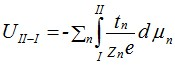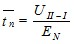ProGasMix FC - Methods

One gas (+ H2O)

You can simply supply one unmixed input gas, wetted, dried, or as a mixture of wet and dry. In this mode, the first parts of the mixer can be used to supply other gases, mixtures, and pH2O values.

Mixture of two gases (+ H2O)

The most commonly applied mixes of two gases are O2 in inert gas, air in inert gas, CO in CO2, and H2 in inert gas. All these typically have the purpose of controlling pO2.
If you are mixing two gases, use G1 as the one generally most dilute, and G2 as the diluent, normally the inert gas or CO2. G1 can then be used undiluted, in a smallest dilution of ca. 1:1, and then continuously more diluted until ca 1:3500, by using the first two stages M1 and M2. If more dilution is required, let G3 = G2 and use also the third stage, obtaining ratios of ca. 1:450,000.

Even higher ratios can be obtained by using a pre-diluted input gas (e.g. air as diluted O2 or 5 % H2 in Ar) or by using the pH2O-stages for mixing the mixture s once more.

Changing the water content (pH2O) in a mixture

The water content of the mixtures can be varied from wet (ca. 3 % H2O at 25°C) to dry (Molecular sieve moisture trap). In addition, the wet can be mixed with dry to cover almost two orders of magnitude of water content down from 3 % (pH2O = 0.03 atm).
This level of water does not influence much on the partial pressure of the other gases in the mixture; from dry to fully wet they decrease by 3 % relatively. For instance, pO2 in dry pure O2 is 1 atm, while pO2 in wet pure O2 is 0.97 atm. Such variations in pH2O with nearly constant pO2 is essential e.g. for studies of proton conductors under oxidizing conditions. Similarly, the pH2O can be changed in CO+CO2 buffer mixtures without affecting pO2 much. (But for H2-based reducing atmospheres pH2O directly affects pO2 and another mode and approach is used, see next section.)

Changing the water content (pH2O) and that of another active component (e.g. pH2) at the same ratio

Assume the mixture going through a wetting stage is H2 in inert gas, e.g. Ar, from a premix and/or from mixing in the first three or four stages. Now, we switch M5DS to G3 and let G3 be Ar. Then the drying stage is not carrying the same mixture as the wetting stage, but instead dried or dry G3 = Ar. In the wetting stage we get wet H2+Ar, i.e. H2O+H2+Ar. When this is mixed with the dry Ar, we are decreasing both the H2O and the H2 contents, but their ratio remains constant. At high temperature equilibrium this means that we are changing pH2 and pH2O, while pO2 remains constant. This is essential for investigations of proton conductors under reducing conditions, in H2+H2O+inert gas atmospheres.

Large gradients; fuel cell and permeation tests

Fuel cells

One typical use of the ProGasMix is in supplying fuel and oxidant to the two sides of a fuel cell test. The oxidant can be diluted and is supplied directly dry or wetted and led to the anode. It may be noted that the fuel in this way can be started and the cathode reduced if necessary in a soft manner by starting with inert gas only at the anode and then mixing in fuel gradually.

One may invert the scheme by supplying air or oxygen instead and mix it with inert gas if necessary.

While dry H2-containing gases may seem the right for the fuel side, this is often too reducing and may embrittle platinum and reduce other components. Better and more stable operation is usually obtained by wetting the H2 mixture fully. The ProGasMix can also wet the oxidant. This may be a good option for work with proton conducting fuel cells of various kinds.

Gas permeation

By supplying different gases over a membrane sample, analysis (e.g. with MS or GC) of the gas composition after the membrane passage on the feed or - in particular - permeate side may be used to obtain the permeation of species in the gases. For measurements of oxygen permeation one may for instance supply O2+N2 mixtures as feed gas on one side. On the permeate side, using Ar, one may analyze the content of N2 and O2. If the membrane is permeable only to oxygen (e.g. through ambipolar diffusion of oxide ions and electrons), the content of N2 may be taken to reflect leakage through pores, cracks or poor seals, and after correcting for this using the fractions of N2 and O2 in the feed gas and assuming macroscopic flow in the leaks, one may obtain the real permeation of oxygen through the membrane material.

If the membrane is at high temperature, a dilute H2+Ar mixture may be used instead, giving a much higher chemical driving force for oxygen, which flux is now measured via the H2O content in the permeate gas.
In a similar manner, a mix of H2 and an inert gas can be supplied as feed gas. The permeate is then typically another inert gas and the levels of H2 and the feed inert gas are measured. Wetting the feed gas is probably a good choice. Wetting the permeate side may give the side effect that any transport of oxygen from the permeate side to the feed side may split water and leave hydrogen, which is then incorrectly assigned to permeation of hydrogen.

Small gradients for transport number measurements

General principles

By establishing a difference in chemical potential over a sample, a driving force for mobile species affected is set up. If the species s is charged a voltage - or electromotive force (EMF) - arises that is proportional to the transport number ts of the species, defined as ts = σs/σtotal.

This emf is measured at open circuit by using high impedance voltmeters, multimeters or electrometers, and is thus often also referred to as an open circuit voltage (OCV). The method we are to describe therefore are called measurements of transport numbers by the OCV of concentration cells, or simply the EMF method.

In its simplest form, the voltage over the cell is derived from so-called Wagner transport theory: The partial current of each charge carrier involved is expressed in terms of the driving force (gradient in electrochemical potential) it experiences, its conductivity, and charge. Then all partial currents are summed to a total current, the expression equaled to zero in the OCV case, and solved with respect to the electrical potential gradient. This is integrated from side I to side II to obtain the voltage over the sample:where UII-I is the voltage between electrodes II and I, tn is the transport number of ion n with charge zn and where μn is the chemical potential of the corresponding neutral atomic species. The expression thus sums up over all ionic species n for which there is a significant gradient and transport number.

In the following, the equation is solved under the assumption that there is a gradient in only one species, and that the gradient is small, so that the transport number can be considered constant, thereafter the integration leads towhere EN is the Nernst voltage. Finally,Software

The software that comes with the ProGasMix will calculate the Nernst voltages involved after you have entered the readings of the flowmeters, and will then give transport numbers based on the voltages measured.
With the ProGasMix one may measure the transport number as a function of the gradient, i.e., the Nernst voltage, and extrapolate back to zero gradient to obtain the transport number closest to the assumption of a small gradient. However, making too small gradients will inevitably lead to immeasurably small voltages and consequently scatter in the transport number curve.

Below we give some examples of types of gradients and how they are obtained in the ProGasMix. However, first we discuss how you can measure small voltages by minimizing or eliminating background offset voltages.

Background (offset) voltage minimization and elimination

Enter here.

Common on EMF transport number measurements

EMF measurements are done by making a basis gas in flowmeters 1-6 that supplies both sides of the sample. The gradient is obtained not by making another gas, by altering the basis gas. These are led to different sides of the sample.

After making the gasses, be sure to let the mixer stabilize and give a stable EMF reading. Then swap the two gases, using the selector valves for the output flowmeters, but letting the rest of the mixer untouched. The EMF should now reverse, and the deviation from a perfect reverse is the offset, which you therefore can obtain and correct for, e.g. by subtracting the two readings (keeping their signs) and dividing by 2. In the above, the selector for Drying stage must be at M3 and M4 (not at Gas 3).

One side of the sample is supplied with the basis gas after setting its pH2O by selecting or mixing wet and dry.

If you want to make a gradient in pO2 (obtaining an EMF proportional to the total ionic transport number), then supply some of the basis mixture to flowmeter 7 and mix in some inert gas (gas 3) by using flowmeter 8. This mixture is now somewhat different from the basis mixture in terms of pO2. Use ratios of typically 50/50, but try also to vary this to see the effect. This mixture is now routed to the second wetting stage and to second drying stage and you now choose the same wet or dry or mix ratio as you did for the basis gas, so that pH2O is the same.

If you want to make a gradient in pH2O (obtaining an EMF proportional to the proton transport number) do the same in flowmeters 1-6, and set its pH2O as before, typically fully wet. Now supply the basis gas to flowmeter 7 as before, but do NOT mix in anything on flowmeter 8 (keeping pO2 the same). Now, in the last wet-dry mixing stage mix in some dry gas so that pH2O becomes somewhat lower than in the other mixture. Again, try something around 50/50 (which on the glass + tantalum ball floweter pair means something like 100+30).

Enter here.

Transients

Enter here.

H/D isotope effects

Enter here.